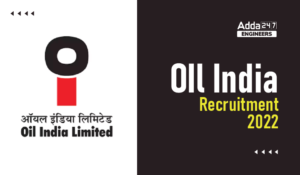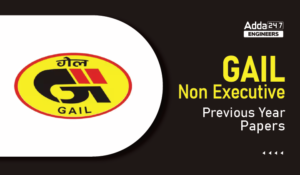Engineering Jobs   »   Quiz: Mechanical Engineering 27th April 2021

# Quiz: Mechanical Engineering 27th April 2021

Quiz: Mechanical Engineering
Exam: AE/JE
Topic: Powerplant Engineering

Each question carries 2 mark
Negative marking: 1/3 mark
Time: 10 Minutes

Q1. How Load factor is defined in Powerplant?
(a) (Average load)/(Rated capacity of plant)
(b) (Average load over a given time interval)/(Peak load during the same time interval

(c) (kwh generated)/(kw installed×operating hours)

Q2. Which among the following relation is correct?
(a) Load factor × Use factor = Capacity factor
(b) Capacity factor × Load factor = Use factor
(c) Use factor × Capacity factor = Load factor
(d) Demand factor × Load factor = Use factor

Q3. What would be the steam rate if turbine produces 200 kJ/kg while pump requires 20 kJ/kg of work?
(a) 0.005
(b) 0.05
(c) 0.0055
(d) 0.5

Q4. What will be the output from turbine if heat rate is 2.2 and steam rate is 0.002 and efficiency is 45.45%?
(a) 2200
(b) 1000
(c) 1100
(d) 1600

Q5. Which among the following causes internal irreversibility in Rankine cycle?
1. Fluid Friction
2. Throttling
3. Mixing
4. Temperature diff. between combustion gas and working fluid
(a) 1,2 and 4 only
(b) 1,3 and 4 only
(c) 1,2 and 3 only
(d) 1,2,3 and 4

Q6. The minimum temperature difference between the condensing steam and cooling water is known as
(a) TTD
(b) Pour points
(c) Pinch points
(d) Any of these

Q7. Which of the following powerplants do not use superheating process?
(a) Fast breed reactor
(b) Pressurized water reactor
(c) Boiling water reactor
(d) Fast neutron reactor

Q8. Gain in cycle efficiency due to employing feedwater heaters follow which law?
(a) Law of increasing return
(b) Law of diminishing return
(c) Either a or b
(d) Neither a nor b

Q9. Which of the following chemicals are injected into feedwater to neutralize the effect of residual dissolved gases?
(a) Sodium benzoate
(b) Sodium sulphite
(c) Sodium carbonate
(d) Potassium carbonate

Q10. Which of the following are properties of coal used in powerplant?
1. Swelling index
2. Weatherability
3. Sulphur content
4. Ash softening temperature
(a) 1,2 and 3 only
(b) 2,3 and 4 only
(c) 1,2 and 4 only
(d) 1,2,3 and 4 only

Solutions
Ans1. (b)
Sol. Load factor is commonly considered as a measure of a powerplant capacity utilization.

Ans2. (a)
Ans 3. (c)
Sol. The capacity of steam plant is often expressed in terms of steam rate or specific steam consumption. It is defined as the rate of steam flow(kg/s) required to produce unit shaft output.
Steam rate = 1/W_net and W_net= W_t-W_p
W_net=200-20
W_net=180
Steam rate =1/180
Steam rate = 0.0055 kg/kWs
Ans 4. (c)
Sol. First find the relation between these variables
η=W_net/W_t
η×W_t=W_net
Taking reciprocal on both sides, we get
1/(η×W_t )=1/W_net
As we know that 1/W_net =Steam rate and 1/η= Heat rate, therefore
W_t= (Heat rate)/(Steam rate)
So, W_t= 2.2/0.002
W_t=1100kJ/kg
Ans 5. (c)
Sol. Internal irreversibility in Rankine cycle is caused due to fluid friction, throttling and mixing while the external irreversibility is caused due to temperature difference between the combustion gases and working fluid on the source side, and the temperature difference between the condensing working fluid and the condenser cooling water on the sink side.
Ans 6. (c)
Sol. The minimum temperature difference between the condensing steam and cooling water is called as pinch point. Pinch point is also used where there is minimum temperature difference between flue gases and steam.
Ans 7. (b)
Sol. Pressurized water reactor or PWR do not use superheat in their operations because superheating causes loss in efficiency of that powerplant.
Ans 8. (b)
Sol. It follows the law of diminishing return because the greatest increment in efficiency is brought by the first feedwater heater. The increment for each additional feedwater heater thereafter successively diminished.
Ans 9. (b)
Sol. Sodium sulphite (〖Na〗2 S(o_3 )) or hydrazine (N_2 H_4) is injected in boiler feed pump to neutralize the effect of dissolved gases.
Ans 10. (d)
Sol. Swelling index, Weatherability, Sulphur content and Ash softening temperature are the properties of coal when it is considered for the use in powerplant.

Sharing is caring!

Thank You, Your details have been submitted we will get back to you.
•DVC Interview Schedule 2022, Download DV...
•ONGC Non Executive Answer Key 2022, Down...
•Oil India Recruitment 2022, Apply Online...
•GAIL Non Executive Previous Year Papers,...
•GAIL Non Executive Previous Year Cutoff ...
•APWD Previous Year Papers, Check here fo...